5. Average maturation cycle

The average maturation cycle is the type that generally takes the company to recover all the money that has invested in the productive process. The number of days that usually the elements that are part of the company finish the production cycle.

For example, a company that sells clothing takes an average of 20 days to sell all of its goods, after paying to its suppliers, and 15 days to charge the clients bills. This company's average maturation cycle is 35 days.

When you calculate it you need to consider that all of its goods do not take the same amount of time to be sold, so to calculate it we use average values.Average maturation cycle worries about when the money came back.

5.1 Sub-periods that formed the average maturation cycle

If we consider an industrial company, we can divide the average maturation cycle into 5 sub-periods:

• Average sourcing period: It represents the days that the generally the raw materials are in stock awaiting to be used. (Pma).

• Average manufacturing period: It is the number of the days that it usually takes to manufacture the products. (Pmf).

• Average selling period: It is he number of days that it takes to sell the manufactured products. (Pmv).

• Average collection period: It is the number of days that it usually takes to collect all the bills from the clients. (Pmc).

• Average payment period: It is the number of days that it usually takes to pay back the bills to the suppliers

5.2 Calculation of the average maturation period

This procedure consists in calculating in the first place the number of rotations that occur in each of the main phases of the period. Once we obtain the number of rotations, we calculate the days that each rotation lasts.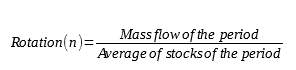Average period for the stock can be calculated is doing an arithmetic average between the initial and final balances of the raw materials, products being manufactured, manufactured products, collection rights and suppliers.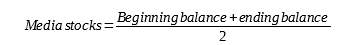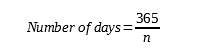Calculation of the average sourcing period:

We consider na as the number of times the average raw materials stock is consumed during te economic exercise.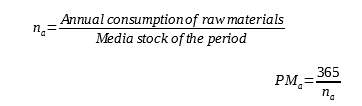Calculation of the average manufacturing period.

We consider nf as the number of times that the manufactured products stock is renewed during the exercise.

Calculation of the average selling period:

We consider nv as the number of times the average manufactured products stock is renewed during the exercise.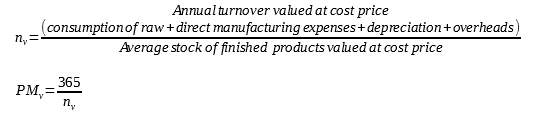Calculation of the average collection period

We consider nc as the number of times the average of the collection rights from clients is renewed during the exercise.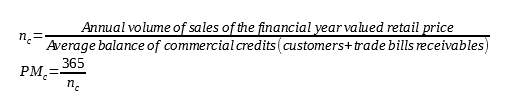Calculation of the average payment period.

We consider np as the number of times the average of the debt is renewed.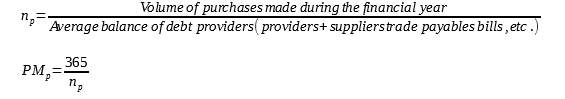To calculate de average maturation period, we can differentiate from average economic maturation period and average financial maturation period.

The average economic maturation period is the time that the entire exploitation cycles lasts, since the raw materials enter until the bills from clients are collected.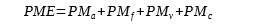The average financial maturation period is the time that the company takes to recover the money that the company has invested in the purchase of materials.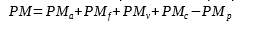5.3 Average maturation period of a commercial company

The companies dedicated to buying and selling and do not have a productive process have 3 sub-periods:

Sourcing sub-period: It is the time that the goods are in stock until they are sold. This sub-period is the equivalent to the average selling period of an industrial company, but the commercial one does not manufacture its own goods.

Collecting sub-period: It is the time the company takes to collect all the bills from clients

Payment sub-period: It is the time the company takes to pay to all its suppliers.

The average financial maturation period can be calculated: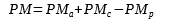5.4 Minimum capital or working capital necessary

In general, it is considered that the working capital must be positive, because there is a part of the elements of the current assets that must be financed by permanent resources (more passive equity non-current).

The working capital needed to finance this part of the current assets is closely related to the financial average maturation period of the company. The longer the time the company takes to recover the investment cycle of exploitation (PMMF) is, the higher also the bottom of maneuver you need, and vice versa.

The working capital is the set of resources that the company needs to fund for the financial period of maturation. Therefore, how much shorter is the maturation period, the lower the working capital necessary.

For the calculation of the minimum required, it can be assumed that the current assets of the company is formed by the stock and collection rights to customers, and current liabilities consists of obligations of payment providers.

Consider the following formula to calculate the average of the above items balance: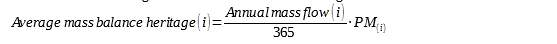The working capital (FM) minimum: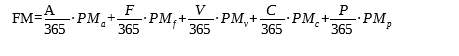A total consumption of the period

F: the production cost of the exercise or mass flow of semi-finished products.

V: cost of sales for the year.

C: annual sales.

Q: total purchases of the period.